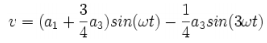Courses

# Generation of DSB-SC Signals Electronics and Communication Engineering (ECE) Notes | EduRev

## Communication Theory

Created by: Machine Experts

## Electronics and Communication Engineering (ECE) : Generation of DSB-SC Signals Electronics and Communication Engineering (ECE) Notes | EduRev

The document Generation of DSB-SC Signals Electronics and Communication Engineering (ECE) Notes | EduRev is a part of the Electronics and Communication Engineering (ECE) Course Communication Theory.
All you need of Electronics and Communication Engineering (ECE) at this link: Electronics and Communication Engineering (ECE)

Generation of DSB-SC Signals

The circuits for generating modulated signals are known as modulators. The basic modulators are Nonlinear, Switching and Ring modulators. Conceptually, the simplest modulator is the product or multiplier modulator which is shown in figure 1-a. However, it is very difficult (and expensive) in practice to design a product modulator that maintains amplitude linearity at high carrier frequencies. One way of replacing the modulator stage is by using a non-linear device. We use the non-linearity to generate a harmonic that contains the product term then use a BPF to separate the term of interest. Figure 3 shows a block diagram of a nonlinear DSBSC modulator. Figure 4 shows a double balanced modulator that use the diode as a non-linear device, then use the BPF to separate the product term.

Sm(t) = ΦDSB-SC(t)= Ac (t) m(t) cos(ωct)

The receiver first generates an exact (coherent) replica (same phase and frequency) of the unmodulated carrier

Sc(t) = Cos(ωct) The coherent carrier is then multiplied with the received signal to give

Sm(t)* Sc(t) = Ac (t) m(t) cos(ωct)* Cos(ωct)

= ½ Ac (t) m(t)+1/2 Ac (t) m(t) cos(2ωct)

The first term is the desired baseband signal while the second is a band-pass signal centered at 2ωc. A low-pass filter with bandwidth equal to that of the m(t) will pass the first term and reject the band-pass component.

Single Side Band (SSB) Modulation:

In DSB-SC it is observed that there is symmetry in the band structure. So, even if one half is transmitted, the other half can be recovered at the received. By doing so, the bandwidth and power of transmission is reduced by half.

Depending on which half of DSB-SC signal is transmitted, there are two types of

1. Lower Side Band (LSB) Modulation

2. Upper Side Band (USB) Modulation

Vestigial Side Band (VSB) Modulation:

The following are the drawbacks of SSB signal generation:

1. Generation of an SSB signal is difficult.

2. Selective filtering is to be done to get the original signal back.

3. Phase shifter should be exactly tuned to 90 0 .

To overcome these drawbacks, VSB modulation is used. It can viewed as a compromise between SSB and DSB-SC.

In VSB

1. One sideband is not rejected fully.

2. One sideband is transmitted fully and a small part (vestige)of the other sideband is transmitted.

The transmission BW is BWv =B + v. where, v is the vestigial frequency band.

FREQUENCY TRANSLATION:

The transfer of signals occupying a specified frequency band, such as a channel or group of channels, from one portion of the frequency spectrum to another, in such a way that the arithmetic frequency difference of signals within the band is unaltered.

FREQUENCY-DIVISION MULTIPLEXING (FDM):

It is a form of signal multiplexing which involves assigning non-overlapping frequency ranges to different signals or to each "user" of a medium.

FDM can also be used to combine signals before final modulation onto a carrier wave. In this case the carrier signals are referred to as subcarriers: an example is stereo FM transmission, where a 38 kHz subcarrier is used to separate the left-right difference signal from the central left-right sum channel, prior to the frequency modulation of the composite signal. A television channel is divided into subcarrier frequencies for video, color, and audio. DSL uses different frequencies for voice and for upstream and downstream data transmission on the same conductors, which is also an example of frequency duplex. Where frequency-division multiplexing is used as to allow multiple users to share a physical communications channel, it is called frequency-division multiple access (FDMA).

NONLINEAR DISTORTION:

It is a term used (in fields such as electronics, audio and telecommunications) to describe the phenomenon of a non-linear relationship between the "input" and "output" signals of - for example - an electronic device.

EFFECTS OF NONLINEARITY:

Nonlinearity can have several effects, which are unwanted in typical situations. The a3 term for example would, when the input is a sine wave with frequency ω, result in an extra sine wave at 3ω, as shown below.In certain situations, this spurious signal can be filtered away because the "harmonic" 3ω lies far outside the frequency range used, but in cable television, for example, third order distortion could cause a 200 MHz signal to interfere with the regular channel at 600 MHz.

Nonlinear distortion applied to a superposition of two signals at different frequencies causes the circuit to act as a frequency mixer, creating intermodulation distortion.

Offer running on EduRev: Apply code STAYHOME200 to get INR 200 off on our premium plan EduRev Infinity!

## Communication Theory

26 videos|29 docs|8 tests

,

,

,

,

,

,

,

,

,

,

,

,

,

,

,

,

,

,

,

,

,

;